# Problem 1

Mtcars
The data was extracted from the 1974 Motor Trend US magazine, and comprises fuel consumption and 10 aspects of automobile design and performance for 32 automobiles (1973–74 models)
1. Create a bar graph showing the mean MPG for different levels of the ‘cyl’ variable.

data11 <- mtcars[, 1:2]
cyl_means <- data.frame(CYL_Number=c(), MPG_Mean=c())
for (cyln in sort(unique(data11[,2]))){
cyl_means <- rbind(cyl_means,
data.frame(CYL_Number=cyln, MPG_Mean=mean(data11[which(data11[,2]==cyln), 1])))
}
print(cyl_means)
##   CYL_Number MPG_Mean
## 1          4 26.66364
## 2          6 19.74286
## 3          8 15.10000

Now the Bar:

barplot(cyl_means[,2], main="Mean of MPG for Number of Cylinders",
xlab="Number or Cylinders", names.arg=c("4", "6", "8"))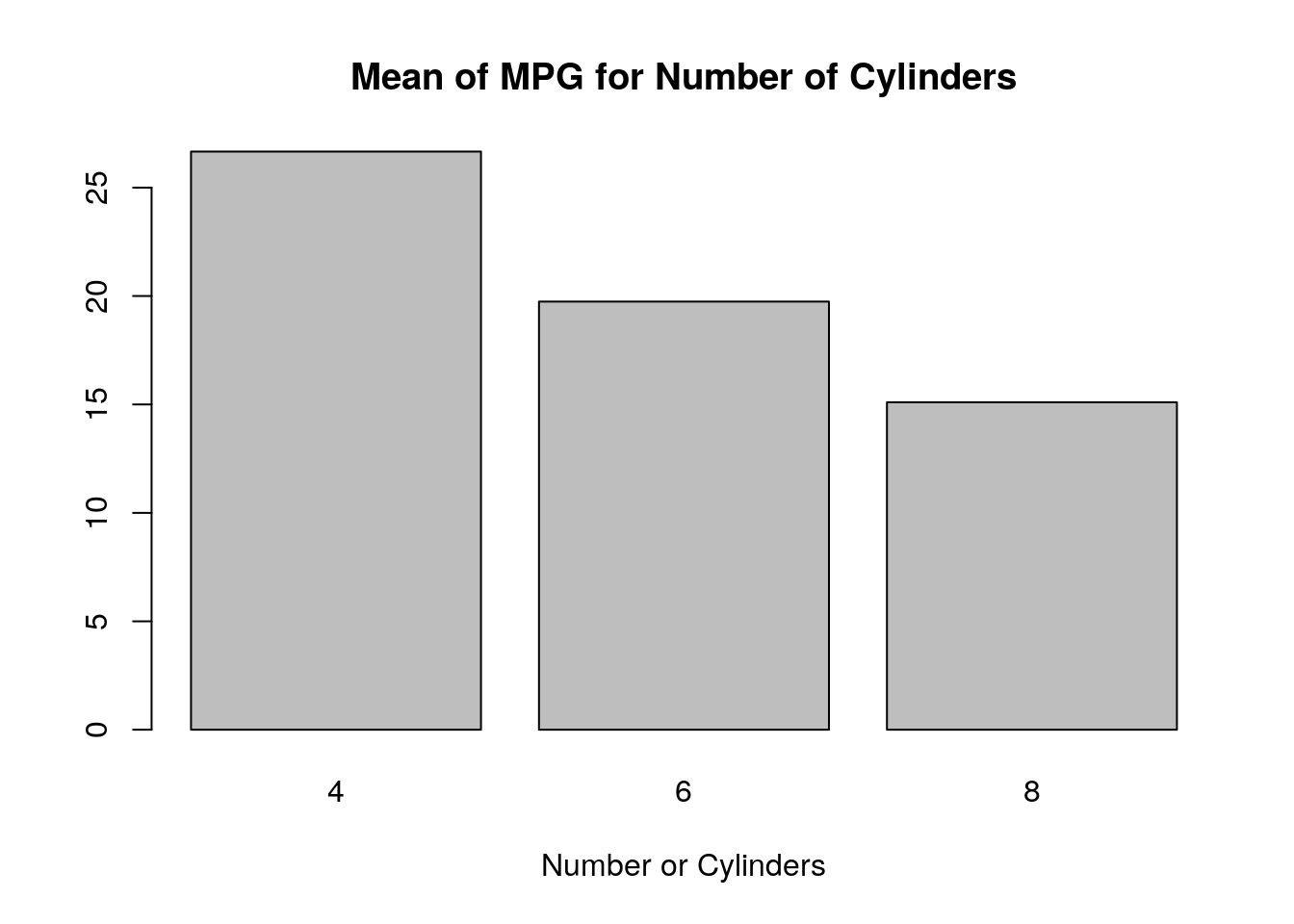2. Create a histogram of the ‘mpg’ variable.

hist(mtcars$mpg, main="MPG distribution", xlab="MPG")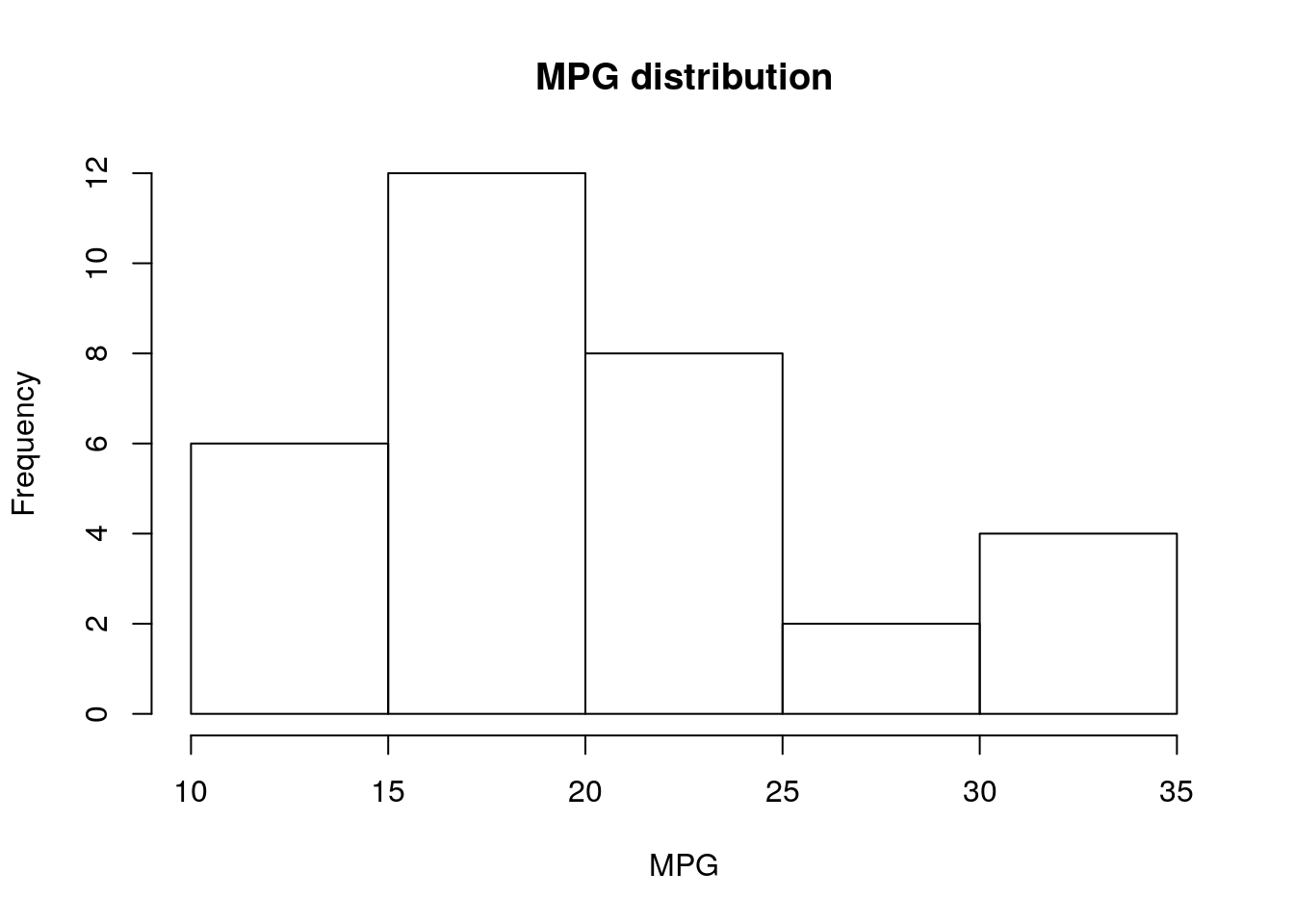3. For these and all other graphs, create a meaningful title, and label and X and Y axes in a way that communicates that the variables are and what are the levels. Done # Problem 2 ToothGrowth contains the result from an experiment studying the effect of vitamin C on tooth growth in 60 Guinea pigs. Each animal received one of three dose levels of vitamin C (0.5, 1, and 2 mg/day) by one of two delivery methods, (orange juice or ascorbic acid (a form of vitamin C and coded as VC). Here is sample code for creating a bar plot of mean-hp by gears and vs in Mtcars dataset : boxplot(hp~(gear*vs), data=mtcars, main="Horse power by gear", xlab="gear/engine combinations", ylab="Horse power", col="gray", border="black", las=1, ylim=c(0,500)) Use the same approach to create a graph plotting tooth-length as function of supplement and does in ToothGrow dataset. boxplot(len~(supp*dose), data=ToothGrowth, main="Tooth Lenght by Supplement", xlab="Supplement/Dose", ylab="Tooth Lenght", col="pink", border="gray", las=1, ylim=c(min(ToothGrowth[,1]),max(ToothGrowth[,1])))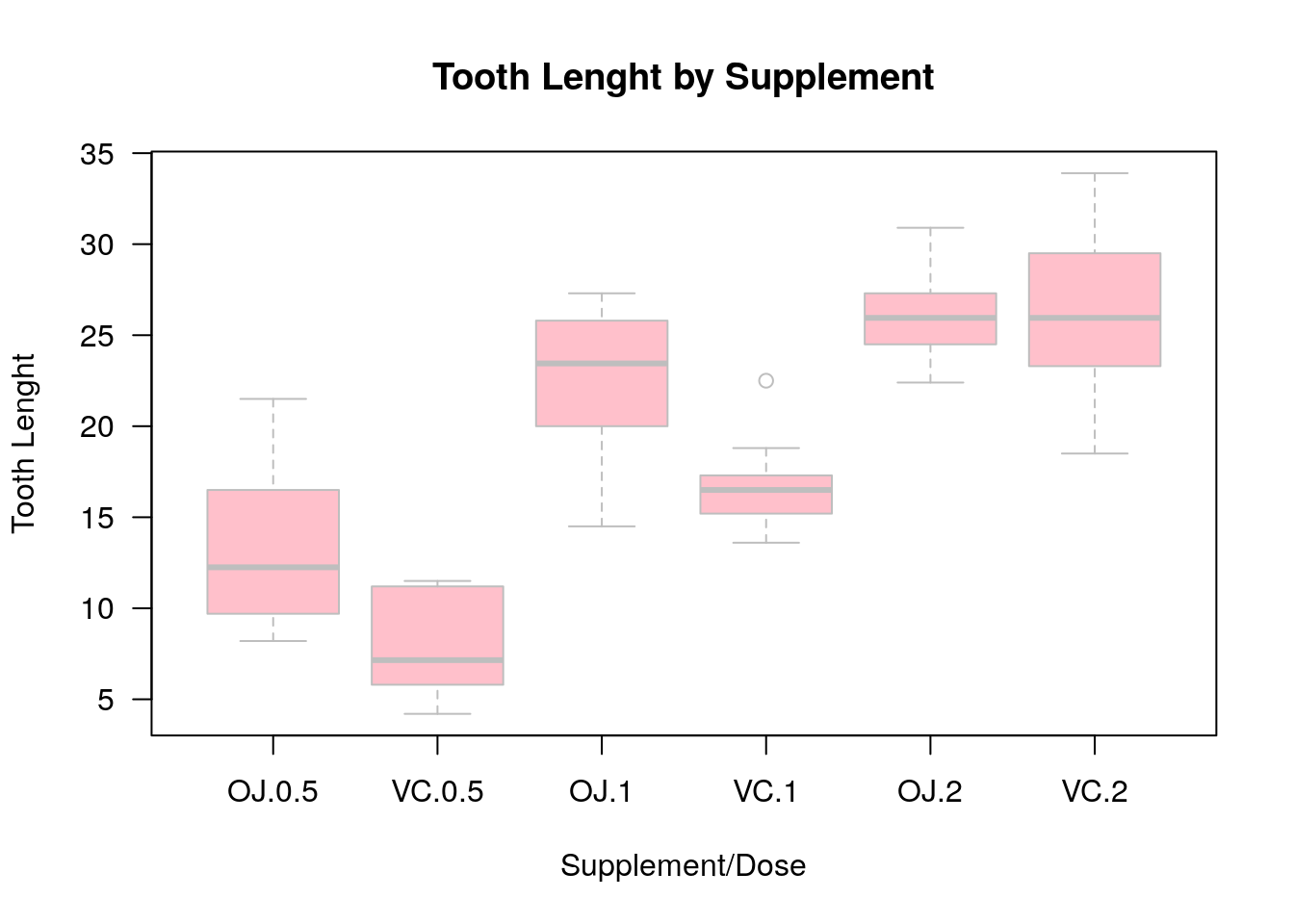# Problem 3 USArrests This data set contains statistics about violent crime rates by us state. 1. What are the means of the Murder and Assault variables? murder_mean <- mean(USArrests$Murder)
assault_mean <- mean(USArrests$Assault) print(paste("Mean of Murder: ", murder_mean), quote = FALSE) ##  Mean of Murder: 7.788 print(paste("Mean of Assault: ", assault_mean), quote = FALSE) ##  Mean of Assault: 170.76 2. What is the standard deviation of the Assault variable? sd_assault <- sd(USArrests$Assault)
print(paste("Standard Deviation of Assault:    ", assault_mean), quote = FALSE)
##  Standard Deviation of Assault:     170.76
3. Create a scatter plot showing the relationship between UrbanPop and Murder. Give it a meaningful title and meaningful X and Y axis labels.

plot(USArrests$Murder, USArrests$UrbanPop,
main="Urban population and Murder Arrest Rate",
xlab="Murder Arrests, per 100,000", ylab="Urban Population %")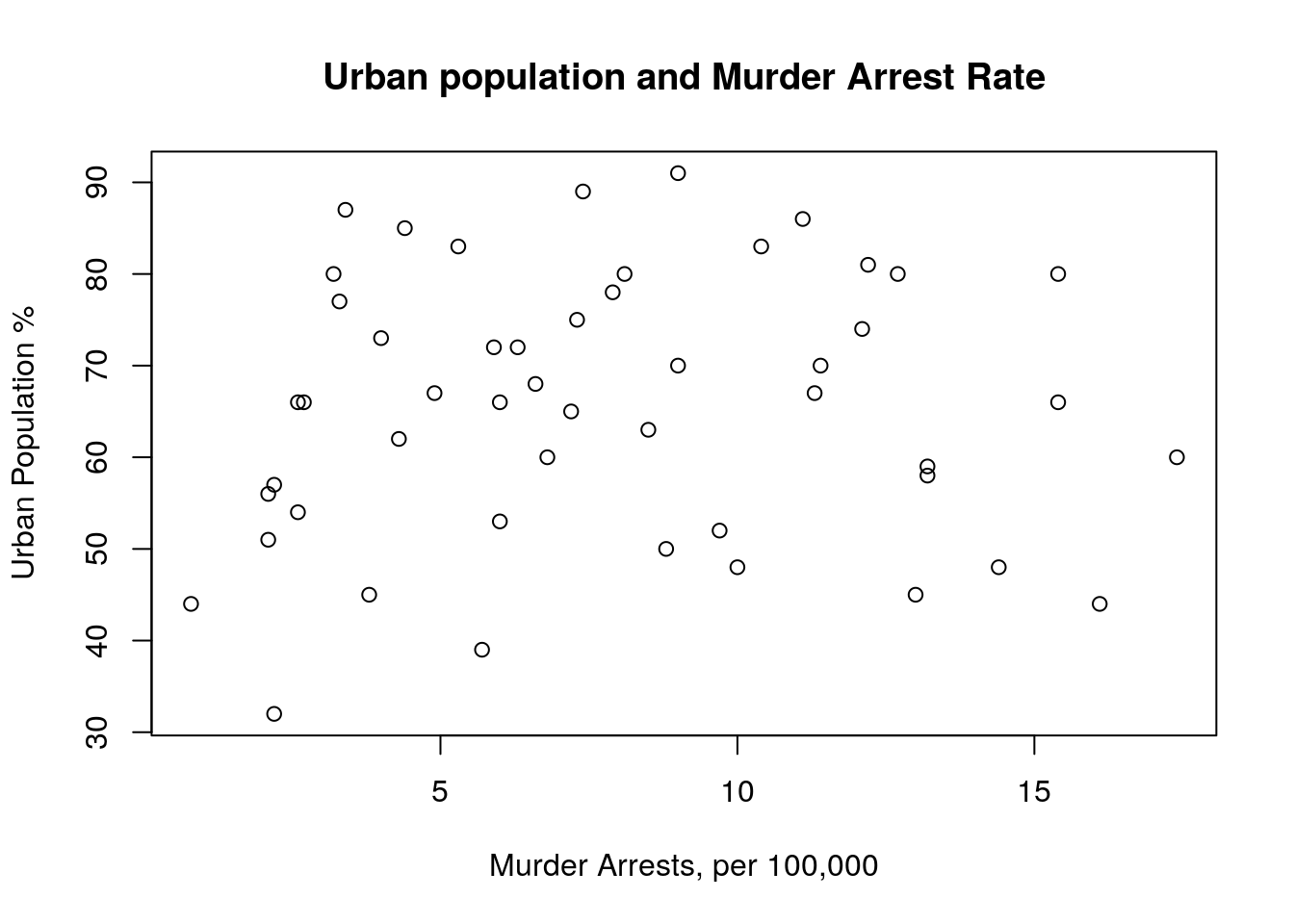4. Create a box and whiskers plot of the Rape variable.

OutVals = boxplot(USArrests$Rape, ylab="Rape Arrest Rate", main="Rape Arrest Rate per State")$out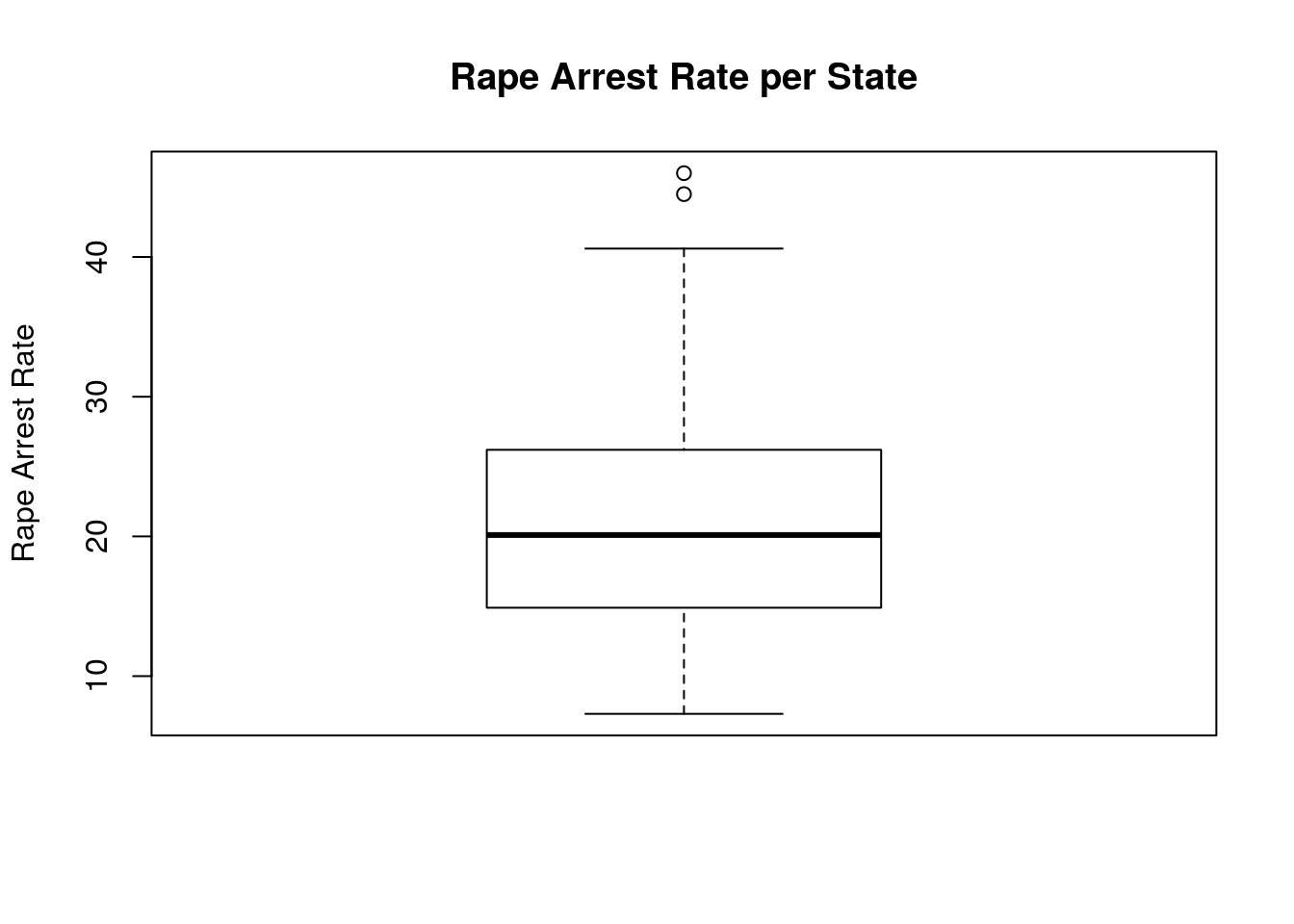How many outliers are there? Use USArrests[order(USArrests$Rape), ] to identify the state(s) that are outliers. # number of outliers: print(paste("Number of outliers: ", length(OutVals)), quote = FALSE) ##  Number of outliers: 2 #Outlier States: out_st <- c() for (i in OutVals){ out_st <- c(out_st, row.names(USArrests)[which(USArrests$Rape == i)])
}
print(out_st)
##  "Alaska" "Nevada"

Go to my HomePage.